# cosecant functions

cosecant functions

There are many angle functions.

Sine, cosine, tangent, cosecant, secant, and cotangent functions are the 6 elementary functions that are used in trigonometry.

Csc, sec and cot are the reciprocal functions of sine, cosine and tangent functions respectively.

The cosecant function is the reciprocal of the sine function.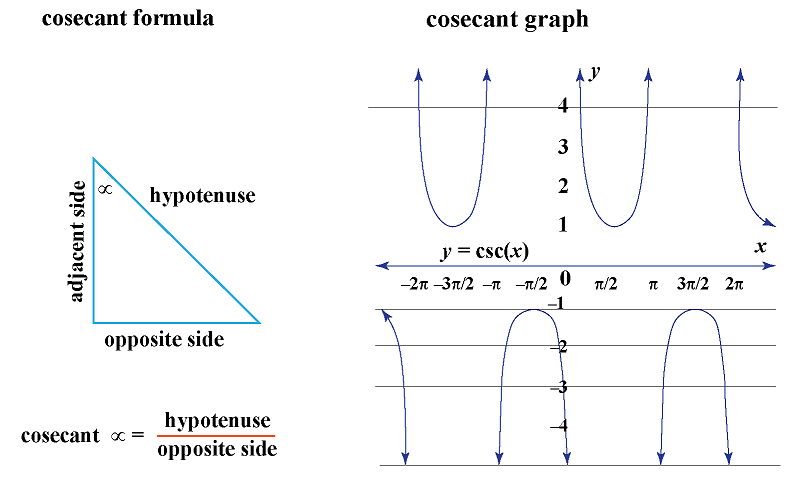In this mini-lesson, let us learn about the properties of the cosecant function and its graph.

## Lesson Plan

 1 What Is a Cosecant Function? 2 Tips and Tricks 3 Important Notes on Cosecant Function 4 Solved Examples on Cosecant Function 5 Interactive Questions on Cosecant Function

## What Is a Cosecant Function?

We have 6 ratios in trigonometry.

The cosecant function is the ratio of the hypotenuse to the opposite side in a right angled triangle.

 $$\csc \theta = \dfrac{1}{sin\theta}$$

### Cosecant Equation

We know that $$\sin \theta = \dfrac{\text{opposite side}}{\text{hypotenuse}}$$, we derive the cosecant equation as follows.

 $$\csc \theta = \dfrac{\text{hypotenuse}}{\text{opposite side}}$$

Consider the 45-45-45 triangle and 30-60-90 triangle and let's determine the cosecant function of the angles.

In the first right triangle, we have the hypotenuse as $$\sqrt{2}$$

\begin{align}\csc \theta &= \dfrac{\text{hypotenuse}}{\text{opposite side}}\\\\\csc 45 &= \dfrac{\sqrt{2}}{1}\\\\&= \sqrt{2}\end{align}

In the second equilateral triangle, we have the hypotenuse as 2

\begin{align}\csc 60 &= \dfrac{\text{hypotenuse}}{\text{opposite side}}\\\\&= \dfrac{2}{\sqrt{3}}\\\\\csc 60 &= \dfrac{2}{1}\\\\&=2\end{align}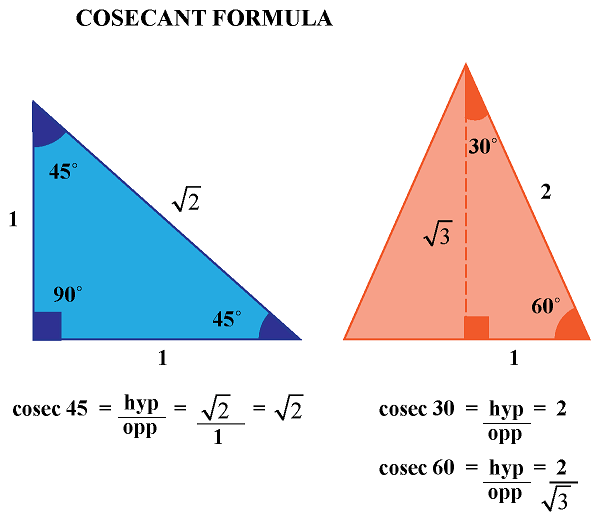## How Do You Graph a Cosecant Function?

Cosecant is a discontinuous graph.

### Sine Graph

By our reasoning of the sine graph, we can quickly graph the cosecant function.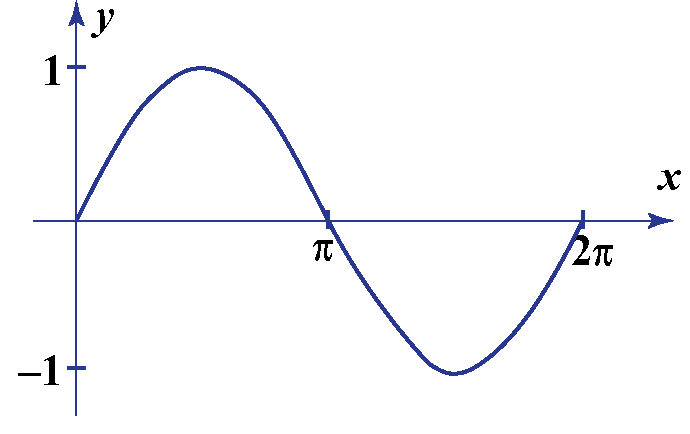### Cosecant Graph

• When $$\theta = 0$$, $$sin \theta = 0$$. $$\because \csc 0 = \text{not defined}$$, we cannot evaluate $$\csc \theta$$.
• When $$\theta = 90$$, $$sin \theta = 1$$, $$\csc \theta = 1$$
• When $$\theta = -90$$, $$sin \theta = - 1$$, $$\csc \theta = -1$$
• When $$\theta = \pm 180$$, $$sin \theta = 0$$. $$\because \csc 0 = \text{not defined}$$
• When $$\theta = \pm 270$$, $$sin \theta = -1$$ , $$\csc \theta = \pm 1$$
• If $$\theta$$ is very small and positive, $$\sin \theta$$ is positive and $$\therefore \csc \theta$$ is large and positive.
• We get vertical asymptotes when the $$\csc \theta$$ cannot be evaluated, that is at $$\sin 0$$ and at $$\sin= n\pi$$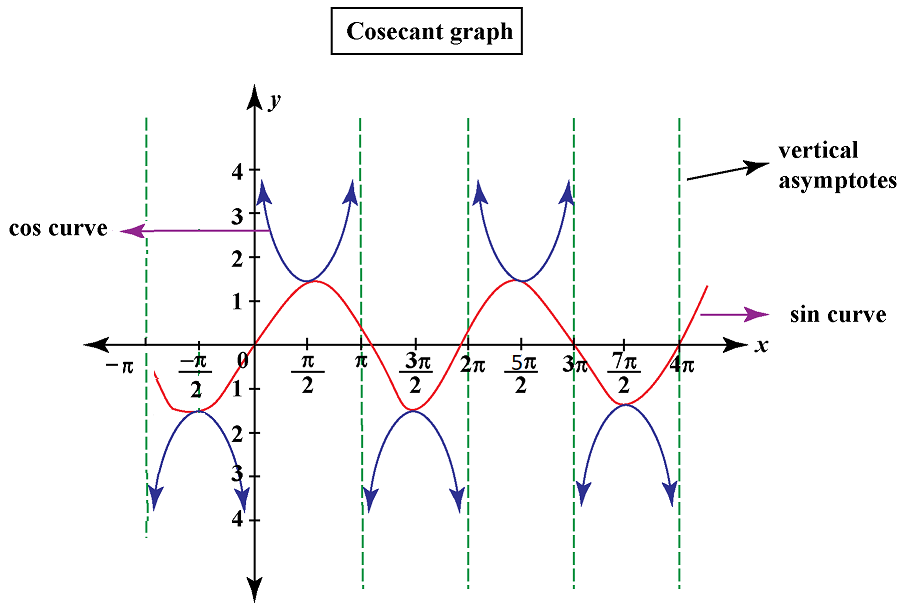Thus, we obtain the cosecant graph.

We have graphed between the intervals $$- \pi$$ and $$4\pi$$.

Note that the vertical asymptotes (the dotted lines in the graph) for the $$\csc$$ function occur at every multiple of $$\pi$$, that is at $$n\pi$$, wherever $$\sin \theta = 0$$.Tips and Tricks
• When sine reaches its maximum value of 1, cosecant will reach its minimum value of 1

• When sine reaches its minimum value of –1, cosecant will reach its maximum value of –1

• When sine is positive $$< 1$$, cosecant will be positive $$> 1$$

• When sine is negative but $$> –1$$, cosecant will be negative but $$< –1$$

## Interpretations - Range, Symmetry, Domain

### Cosecant Domain and Range

The domain of the function $$y=\csc(\theta) = \dfrac{1}{sin(\theta)}$$ is all real numbers except the values where $$\sin(\theta) = 0$$ that is, the values $$n \pi$$ for all integers $$n$$.

Domain  Range
The set of $$\theta$$ values for which $$\csc$$ function is defined are all the real numbers where $$\theta \neq 0$$ $$(-\infty,-1)$$ or $$(1 , \infty)$$
$$\theta \in R, \theta \neq 0, 180, 360, ....$$ $$csc \theta \leq−1$$ or $$csc \theta \geq1$$

### Cosecant Symmetry

The cosecant function is an odd function, that is, the function is symmetrical around the origin.

Let $$f(x) = \csc (x)$$.

Its symmetry is $$\csc (-x)$$.

There are no x-intercepts in the cosecant graph. It is symmetrical about the origin.

Observe the following graph which is drawn on an interval of $$-2\pi, 2\pi$$.

We see that the graph is symmetrical when rotated at $$180^{\circ}$$.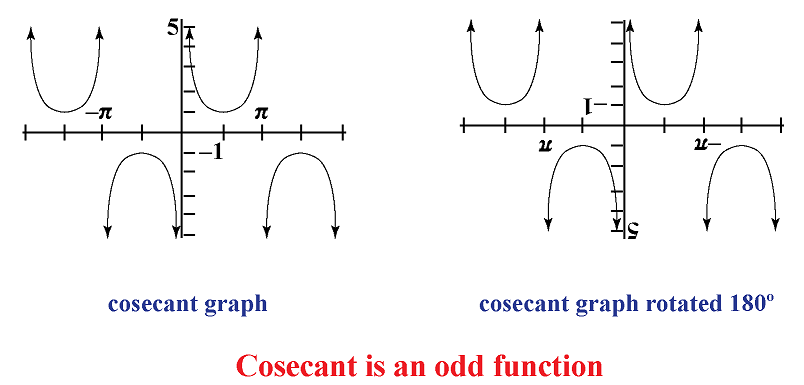### Csc Calculator

Key in the value of the angle you are looking for in the csc calculator below and find the cosecant value of the angle.

## Solved Examples

 Example 1

Find the value of $$\csc \alpha$$ and $$\csc\beta$$.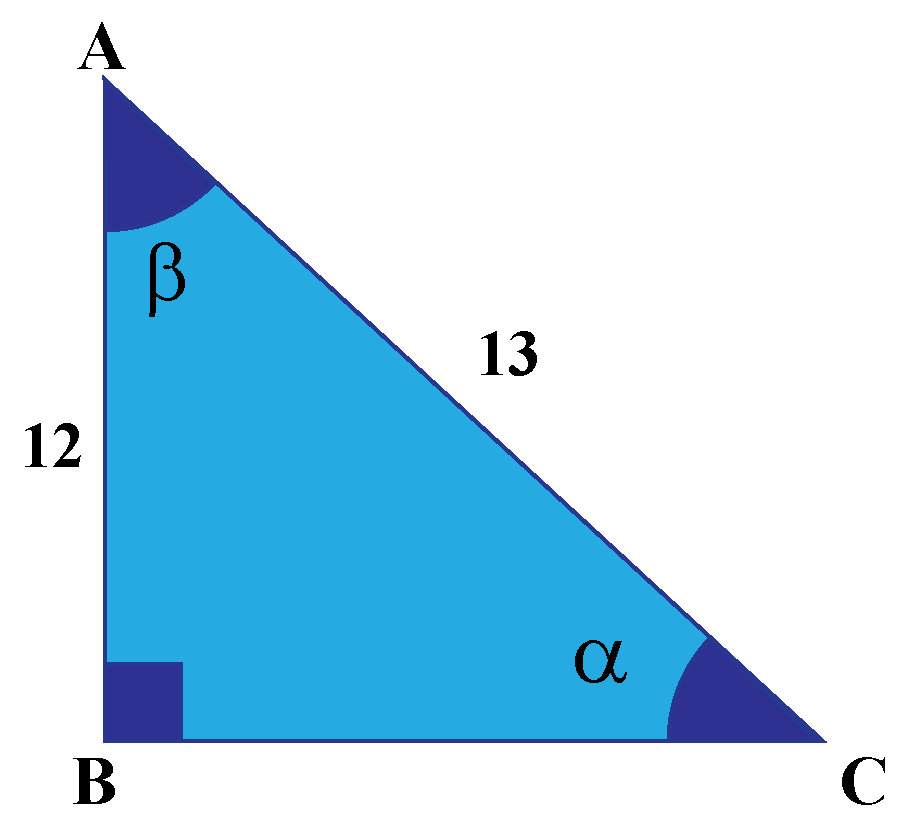Solution

The hypotenuse (h) = 13

\begin{align}\csc \alpha &=\dfrac{\text{hypotenuse}}{\text{opposite side}}\\\\&=\dfrac{13}{12}\\\\&= 1.08\\\\\csc \beta &=\dfrac{\text{hypotenuse}}{\text{opposite side}}\\\\\text{opposite side} &= \sqrt{13^2 -12^2}\\\\&= \sqrt{169-144} \\\\&=\sqrt{25}\\\\&= 5\\\\\csc\beta&=\dfrac{13}{5}\\\\&= 2.6\end{align}

 $$\therefore$$ $$\csc \alpha = 1.08$$ and $$\csc\beta= 2.6$$
 Example 2

Why is the $$\csc$$ of an angle always greater than 1?

Solution

The cosecant function is the relationship between the hypotenuse and the opposite side in a right triangle.

We know that $$\csc \theta = \dfrac{\text{hypotenuse}}{\text{opposite side}}$$.

The numerator always is larger than the denominator as the hypotenuse is the longest side.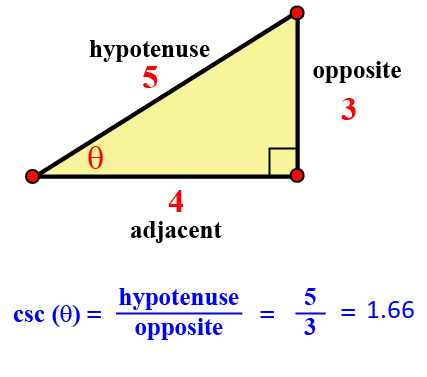Thus, the cosecant function is greater than 1

## Interactive Questions

Here are a few activities for you to practice. Select/Type your answer and click the "Check Answer" button to see the result.

## Let's Summarize

The mini-lesson targeted the fascinating concept of Cosecant Function. The math journey around Cosecant Function started with what a student already knew and went on to creatively crafting a fresh concept in the young minds. Done in a way that not only it is relatable and easy to grasp, but also will stay with them forever.

At Cuemath, our team of math experts is dedicated to making learning fun for our favorite readers, the students!

Through an interactive and engaging learning-teaching-learning approach, the teachers explore all angles of a topic.

Be it worksheets, online classes, doubt sessions, or any other form of relation, it’s the logical thinking and smart learning approach that we, at Cuemath, believe in.

## FAQs on Cosecant Function

### 1. What is a cosecant line?

The line segment in the unit circle as shown below is the cosecant line.

AD is the cosecant line in this unit circle.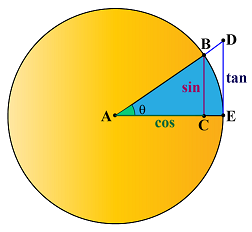### 2. What is the difference between secant and cosecant?

Secant is the reciprocal of cosine function, whereas cosecant is the reciprocal of sine function.

### 3. Do secant have asymptotes?

Yes, secant and cosecant functions have vertical asymptotes.

### 4. How do you write an equation for a cosecant graph?

$$\csc (x) = \dfrac{1}{\sin(x)}$$

### 5. What is CSC of an angle?

Cosecant of an angle is the ratio of the hypotenuse to the opposite side in a right-angled triangle.

More Important Topics
Numbers
Algebra
Geometry
Measurement
Money
Data
Trigonometry
Calculus
More Important Topics
Numbers
Algebra
Geometry
Measurement
Money
Data
Trigonometry
Calculus
Learn from the best math teachers and top your exams

• Live one on one classroom and doubt clearing
• Practice worksheets in and after class for conceptual clarity
• Personalized curriculum to keep up with school